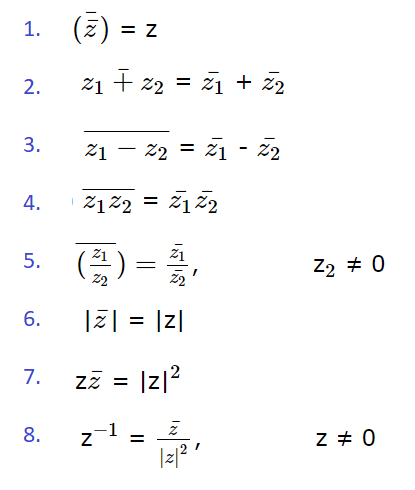# Complex Conjugate: Definition, Properties

A complex conjugate z, has one real part and one imaginary part; the parts have the same magnitude but different signs. In other words, the conjugate of a complex number is the same number but a reversed sign for the imaginary part.

Generally, speaking, the complex conjugate of a + bi is abi (where a and b are two real numbers).

A few examples:

• Conjugate of z = 5 + 3i is z = 5 – 3i
• Conjugate of z = -6 – i is z = -6 + i
• Conjugate of z = 9i is z = -9i

A complex conjugate negates the imaginary part, so geometrically it is a transformation of the complex plane where all points are reflected over the real axis. All points above and below the axis are exchanged; in other words, you can find the complex conjugate of any complex number geometrically by reflecting z across the real axis.

## Complex Conjugate Properties and Rules

The following properties and rules apply to complex conjugates:## Usefulness of the Complex Conjugate

The complex conjugate is very useful because if you multiply any complex number by its conjugate, you end up with a real number :
(a + jb)(a – jb) = a2 – j2b2 = a2 + b2.

It also gives us another way to interpret reciprocals. A complex number multiplied by its conjugate is the square of its absolute value (or complex modulus):
z · z = |z|2.
Therefore:
Geometrically, 1/|z| and z are on the same ray from the origin, but 1/|z| is a quarter of the length , as the following image shows:## References

Complex conjugate image: Oleg Alexandrov, CC BY-SA 3.0 , via Wikimedia Commons.
 Multiplication and Division. Retrieved November 9, 2021 from: http://hyperphysics.phy-astr.gsu.edu/hbase/cmplx2.html
 Joyce, D. Dave’s Short Course on Complex Numbers. Reciprocals, conjugates, and division. Retrieved November 9, 2021 from: https://www2.clarku.edu/faculty/djoyce/complex/div.html

CITE THIS AS:
Stephanie Glen. "Complex Conjugate: Definition, Properties" From StatisticsHowTo.com: Elementary Statistics for the rest of us! https://www.statisticshowto.com/complex-conjugate/